### 4.5Comparison of Improved Implication and Reprogrammable Gates

Fig. 4.21 compares the average error probabilities () of different logic operations using the CC-IMP gate ((N)IMP operation) and a two-input reprogrammable logic gate (AND, OR, NAND, and NOR operations) as a function of TMR ratio with optimized circuit parameters (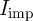,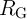, and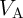) at each point. The MTJ device parameters are given as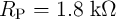,,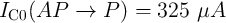, and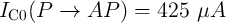. It illustrates that the error decreases exponentially with increasing TMR ratio and for the same device characteristics, the implication gate exhibits a more reliable logic behavior as compared to the reprogrammable gate. It has to be mentioned that these results are obtained in the MTJ thermally-activated switching regime (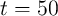ns), which is rather slow for logic applications. However, as the MTJ-based logic mainly relies on a current modulation required for STT switching, the superior reliability of the implication gate is independent of the switching regime as it is demonstrated below in Fig. 4.22.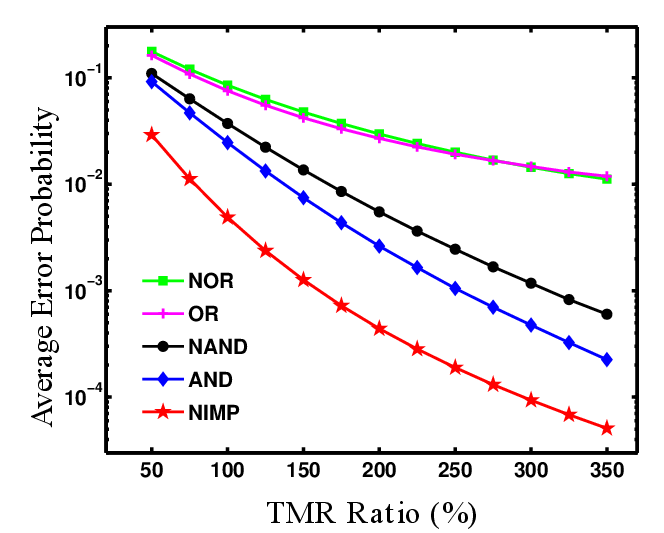Figure 4.21.: Average error probabilities for the implication and two-input reprogrammable logic gates as a function of the TMR ratio.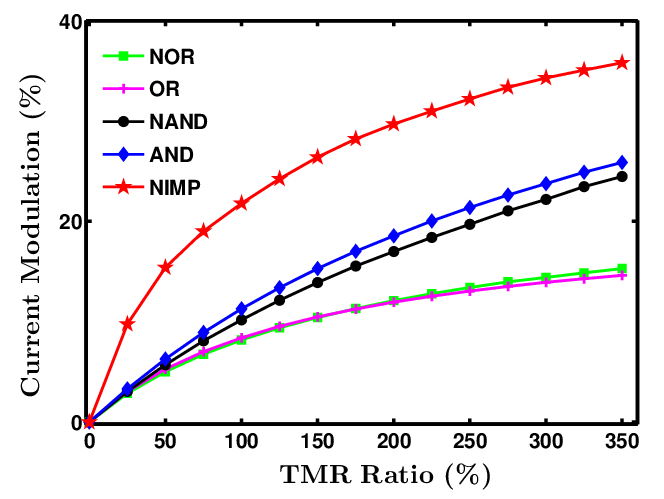Figure 4.22.: Maximum current modulation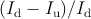in implication and two-input reprogrammable logic gates as a function of the TMR ratio.

Fig. 4.22 compares the maximum current modulations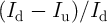for each MTJ-based operation as a function of the TMR ratio.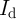is the minimum current required for a desired resistance switching and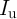is the maximum current which can enforce an undesired resistance switching. For example, in the implication gate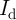(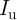) is the current flowing through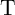(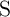) in State 1. For the reprogrammable-based AND operation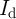(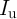) is the current flowing through the output MTJ in State 4 (State 2 and State 3). As shown in Fig. 4.22, the implication gate allows higher current modulations compared to the highest modulation by the reprogrammable-based AND operation. Therefore, it opens a wider gap between desired and undesired switching events and thus inherently enables a more reliable logic behavior. In fact, with the implication logic gate,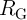provides one more degree of freedom for the circuit parameters’ optimization. Therefore, the basic logic operation by the implication logic gate exhibits five times more reliable behavior as compared to the most reliable operation (AND) implemented by the reprogrammable gate (Fig. 4.21). The record TMR ratio of 604%  makes the MgO-based MTJ a major candidate for STT-operated magnetoresistive random-access memories (STT-MRAMs) and promises highly reliable MTJ-based logic architectures.

In order to preform a fair comparison, we assume the same MTJ device characteristics for both logic gates and determine the minimum possible error probabilities (corresponding to optimized circuit parameters as shown in Fig. 4.15b and Fig. 4.20) for implementing the same binary Boolean functions using the implication and the reprogrammable logic gates. For implication-based logic, appropriate sequential steps of NIMP and TRUE operations must be executed to perform a specific logic function as designed before. With the reprogrammable gate, a network of basic logic operations including AND, OR, NAND, and NOR has to be constructed. Each basic operation includes a preset (TRUE or FALSE) and a conditional switching event (Table 4.1 and Table 4.2)). We define the average error probability of a complex Boolean logic function (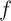) implemented by using a sequence of the basic logic operations as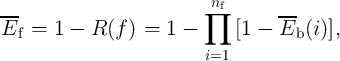(4.27)

where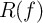is the reliability of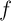,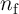indicates the total number of required basic logic operations for implementing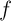, and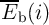corresponds to the average error probability of the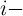th basic logic operation. Since by applying high enough voltage/current highly reliable TRUE and FALSE operations can be executed, we suppose that the error probability of a TRUE or FALSE operation is negligible compared to the error probabilities of conditional switching events in both implication and reprogrammable gates. Therefore,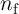is equal to the total number of the conditional switching events required for performing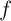based on either implication or reprogrammable gates.

 Table 4.3.: Average error probabilities for 7 distinct binary Boolean functions based on the implication and reprogrammable logic architectures for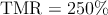.

 Reprogrammable ReprogrammableImplication AND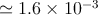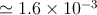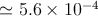OR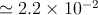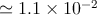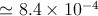NAND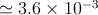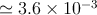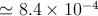NOR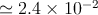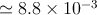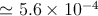NOT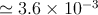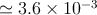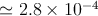IMP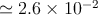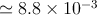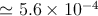NIMP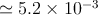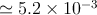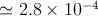As an example, performing an implication-based NOR operation requires three sequential steps including one TRUE and two NIMP operations as shown in Eq. 3.2. Therefore, for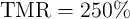(Fig. 4.21),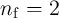and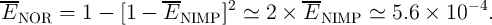(4.28)

With the reprogrammable gate, one can directly perform NOR in two steps including one FALSE and one conditional switching as shown in Table 4.2), for which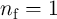and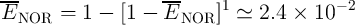(4.29)

A more efficient way to reduce the error probability with the reprogrammable gate is designing and performing the logic function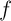only based on the more reliable AND and NAND operations (Fig. 4.20 and Fig. 4.21). Therefore, a reprogrammable-based NOR operation can be indirectly executed as a combination of two NAND and one AND operations (in a sequential manner) for which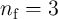and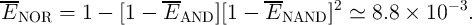(4.30)

This kind of design (shown as reprogrammablein Table 4.3) exhibits a more reliable behavior as compared to the direct realization of the reprogrammable-based NOR operation. However, its error is still about one order of magnitude higher than the implication-based implementation. This shows that the implication logic has a great potential to form a highly reliable MTJ-based logic framework.

Table 4.3 shows the average error probability (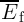) of different Boolean functions for implication and reprogrammable logic gates based on Eq. 4.27 and the error values shown in Fig. 4.21 for TMR=250%. Reprogrammableshows the results for the designs with minimized error probabilities based on AND and NAND operations. For the sake of completeness, performing the NOT operation requires one TRUE and one NIMP (NAND) operation using the implication (reprogrammable ) logic architecture.

 TRUE : t = 1 NIMP : t → s ≡{t′ = t.s ≡ NOT s} (4.31) TRUE : a2 = 1 Preset : b1 = 0 NAND : b1 ←a1.a2 ≡ NOT a1 (4.32)